# 14-240/Tutorial-November11

## Boris

#### Useful Definitions

Let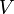$V$ be a finite dimensional vector space over a field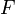$F$,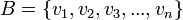$B = \{v_1, v_2, v_3, ..., v_n\}$ be an ordered basis of$V$ and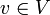$v \in V$. Then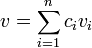$v = \displaystyle\sum_{i=1}^{n} c_iv_i$ where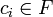$c_i \in F$. Then the coordinate vector of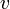$v$ relative to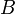$B$ is the column vector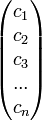$\begin{pmatrix}c_1\\c_2\\c_3\\...\\c_n\end{pmatrix}$.

Let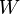$W$ be a finite dimensional vector space over the same field$F$ and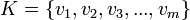$K = \{v_1, v_2, v_3, ..., v_m\}$ be an ordered basis of$W$. Define a linear transformation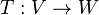$T:V \to W$. Then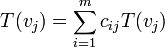$T(v_j) = \displaystyle\sum_{i=1}^{m} c_{ij}T(v_j)$ where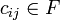$c_{ij} \in F$. Then the matrix representation of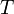$T$ in the ordered bases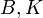$B, K$ is the matrix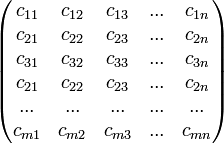$\begin{pmatrix}c_{11} & c_{12} & c_{13} & ... & c_{1n}\\c_{21} & c_{22} & c_{23} & ... & c_{2n}\\c_{31} & c_{32} & c_{33} & ... & c_{3n}\\c_{21} & c_{22} & c_{23} & ... & c_{2n}\\... & ... & ... & ...& ... \\c_{m1} & c_{m2} & c_{m3} & ... & c_{mn}\end{pmatrix}$.

#### Boris's Problems

Let$B$ be the standard ordered basis of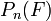$P_n(F)$ and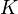$K$ be the standard ordered basis of$F$.

Q1. What is the coordinate vector of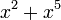$x^2 + x^5$ relative to$B$?

Q2. Let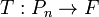$T:P_n \to F$ be a linear transformation that is defined by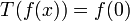$T(f(x)) = f(0)$. What is the matrix representation of$T$ in$B, K$?